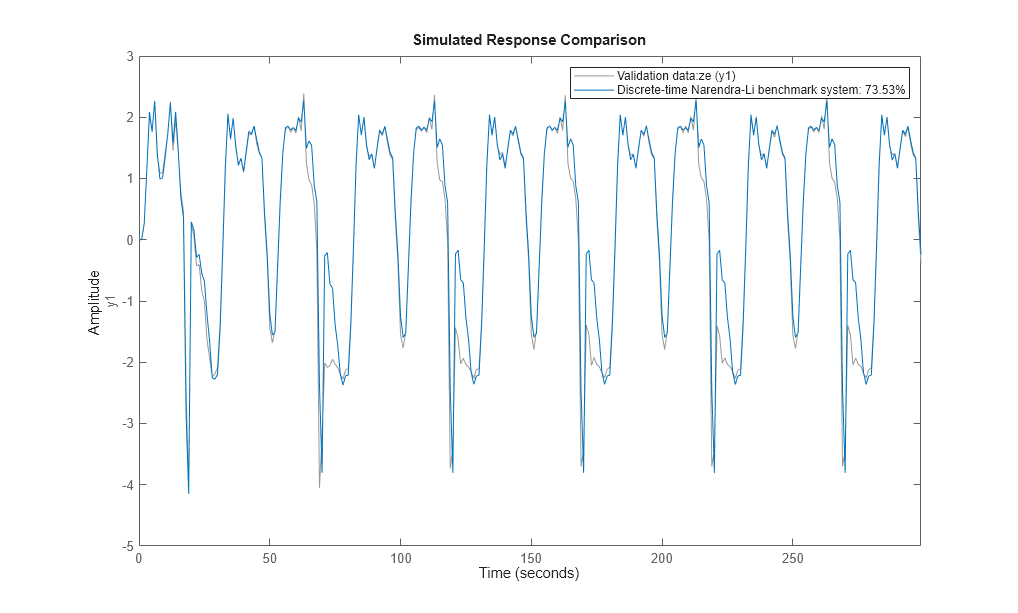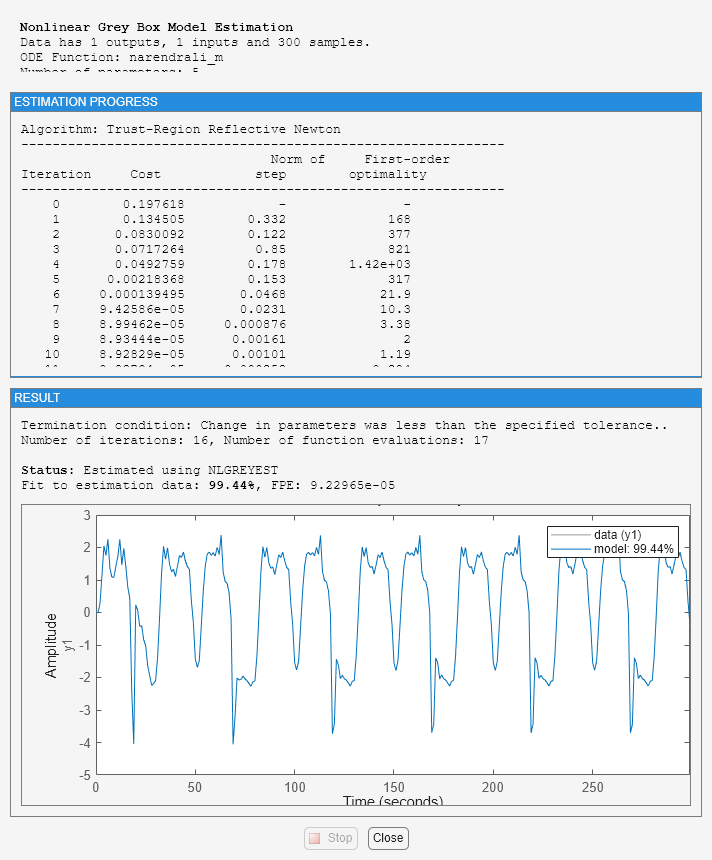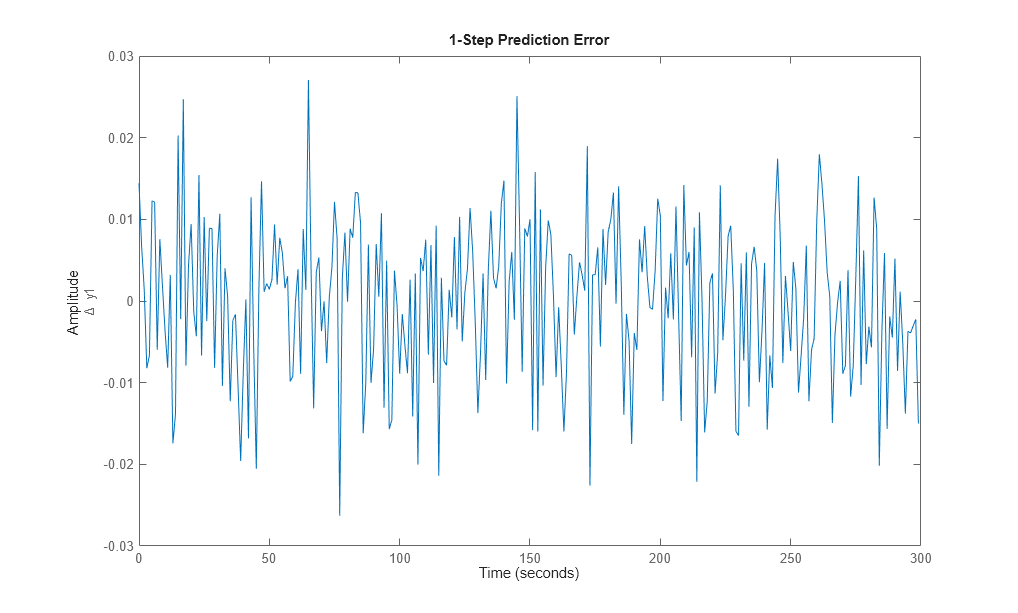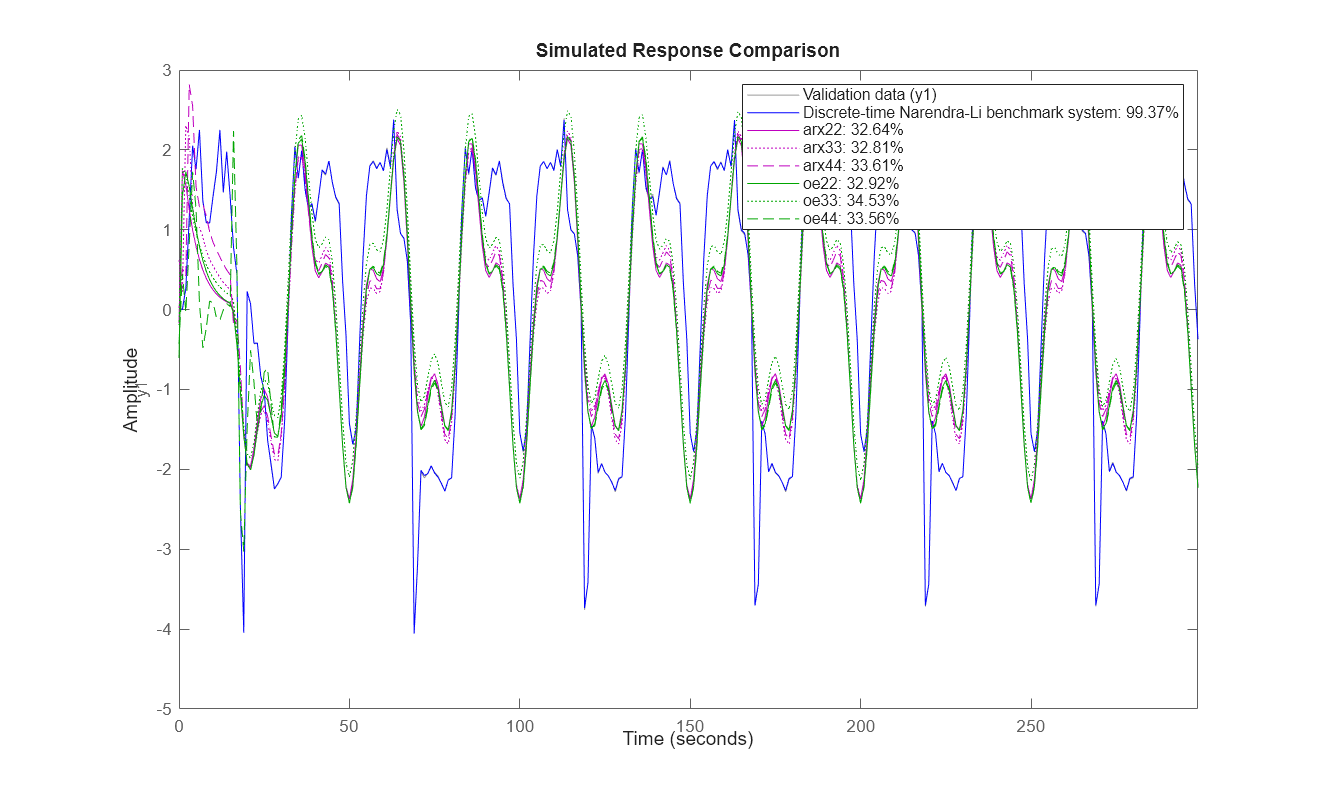# Narendra-Li Benchmark System: Nonlinear Grey Box Modeling of a Discrete-Time System

This example shows how to identify the parameters of a complex yet artificial nonlinear discrete-time system with one input and one output. The system was originally proposed and discussed by Narendra and Li in the article

``` K. S. Narendra and S.-M. Li. "Neural networks in control systems". In Mathematical Perspectives on Neural Networks (P. Smolensky, M. C. Mozer, and D. E. Rumelhard, eds.), Chapter 11, pages 347-394, Lawrence Erlbaum Associates, Hillsdale, NJ, USA, 1996.```

and has been considered in numerous discrete-time identification examples.

### Discrete-Time Narendra-Li Benchmark System

The discrete-time equations of the Narendra-Li system are:

``` x1(t+Ts) = (x1(t)/(1+x1(t)^2)+p(1))*sin(x2(t)) x2(t+Ts) = x2(t)*cos(x2(t)) + x1(t)*exp(-(x1(t)^2+x2(t)^2)/p(2)) + u(t)^3/(1+u(t)^2+p(3)*cos(x1(t)+x2(t))```
` y(t) = x1(t)/(1+p(4)*sin(x2(t))+p(5)*sin(x1(t)))`

where x1(t) and x2(t) are the states, u(t) the input signal, y(t) the output signal and p a parameter vector with 5 elements.

### IDNLGREY Discrete-Time Narendra-Li Model

From an IDNLGREY model file point of view, there is no difference between a continuous- and a discrete-time system. The Narendra-Li model file must therefore return the state update vector dx and the output y, and should take t (time), x (state vector), u (input), p (parameter(s)) and varargin as input arguments:

``` function [dx, y] = narendrali_m(t, x, u, p, varargin) %NARENDRALI_M A discrete-time Narendra-Li benchmark system.```
``` % Output equation. y = x(1)/(1+p(4)*sin(x(2)))+x(2)/(1+p(5)*sin(x(1)));```
``` % State equations. dx = [(x(1)/(1+x(1)^2)+p(1))*sin(x(2)); ... % State 1. x(2)*cos(x(2))+x(1)*exp(-(x(1)^2+x(2)^2)/p(2)) ... % State 2. + u(1)^3/(1+u(1)^2+p(3)*cos(x(1)+x(2))) ... ];```

Notice that we have here chosen to condense all 5 parameters into one parameter vector, where the i:th element is referenced in the usual MATLAB way, i.e., through p(i).

With this model file as a basis, we next create an IDNLGREY object reflecting the modeling situation. Worth stressing here is that the discreteness of the model is specified through a positive value of Ts (= 1 second). Notice also that Parameters only holds one element, a 5-by-1 parameter vector, and that SETPAR are used to specify that all the components of this vector are strictly positive.

```FileName = 'narendrali_m'; % File describing the model structure. Order = [1 1 2]; % Model orders [ny nu nx]. Parameters = {[1.05; 7.00; 0.52; 0.52; 0.48]}; % True initial parameters (a vector). InitialStates = zeros(2, 1); % Initial value of initial states. Ts = 1; % Time-discrete system with Ts = 1s. nlgr = idnlgrey(FileName, Order, Parameters, InitialStates, Ts, 'Name', ... 'Discrete-time Narendra-Li benchmark system', ... 'TimeUnit', 's'); nlgr = setpar(nlgr, 'Minimum', {eps(0)*ones(5, 1)}); ```

A summary of the entered Narendra-Li model structure is obtained through the PRESENT command:

```present(nlgr); ```
``` nlgr = Discrete-time nonlinear grey-box model defined by 'narendrali_m' (MATLAB file): x(t+Ts) = F(t, u(t), x(t), p1) y(t) = H(t, u(t), x(t), p1) + e(t) with 1 input(s), 2 state(s), 1 output(s), and 5 free parameter(s) (out of 5). Inputs: u(1) (t) States: Initial value x(1) x1(t) xinit@exp1 0 (fixed) in [-Inf, Inf] x(2) x2(t) xinit@exp1 0 (fixed) in [-Inf, Inf] Outputs: y(1) (t) Parameters: Value p1(1) p1 1.05 (estimated) in ]0, Inf] p1(2) 7 (estimated) in ]0, Inf] p1(3) 0.52 (estimated) in ]0, Inf] p1(4) 0.52 (estimated) in ]0, Inf] p1(5) 0.48 (estimated) in ]0, Inf] Name: Discrete-time Narendra-Li benchmark system Sample time: 1 seconds Status: Created by direct construction or transformation. Not estimated. More information in model's "Report" property. ```

### Input-Output Data

Two input-output data records with 300 samples each, one for estimation and one for validation purposes, are available. The input vector used for both these records is the same, and was chosen as a sum of two sinusoids:

```u(t) = sin(2*pi*t/10) + sin(2*pi*t/25) ```

for t = 0, 1, ..., 299 seconds. We create two different IDDATA objects to hold the two data records, ze for estimation and zv for validation purposes.

```load(fullfile(matlabroot, 'toolbox', 'ident', 'iddemos', 'data', 'narendralidata')); ze = iddata(y1, u, Ts, 'Tstart', 0, 'Name', 'Narendra-Li estimation data', ... 'ExperimentName', 'ze'); zv = iddata(y2, u, Ts, 'Tstart', 0, 'Name', 'Narendra-Li validation data', ... 'ExperimentName', 'zv'); ```

The input-output data that will be used for estimation are shown in a plot window.

```figure('Name', ze.Name); plot(ze); ```Figure 1: Input-output data from a Narendra-Li benchmark system.

### Performance of the Initial Narendra-Li Model

Let us now use COMPARE to investigate the performance of the initial Narendra-Li model. The simulated and measured outputs for `ze` and `zv` are generated, and fit to `ze` is shown by default. Use the plot context menu to switch the data experiment to `zv`. The fit is not that bad for either dataset (around 74 %). Here we should point out that COMPARE by default estimates the initial state vector, in this case one initial state vector for `ze` and another one for `zv`.

```compare(merge(ze, zv), nlgr); ```Figure 2: Comparison between true outputs and the simulated outputs of the initial Narendra-Li model.

```%%4 % By looking at the prediction errors obtained via PE, we realize that the % initial Narendra-Li model shows some systematic and periodic differences % as compared to the true outputs. pe(merge(ze, zv), nlgr); ```Figure 3: Prediction errors obtained with the initial Narendra-Li model. Use context menu to switch between experiments.

### Parameter Estimation

In order to reduce the systematic discrepancies we estimate all 5 parameters of the Narendra-Li model structure using NLGREYEST. The estimation is carried out based on the estimation data set ze only.

```opt = nlgreyestOptions('Display', 'on'); nlgr = nlgreyest(ze, nlgr, opt); ```### Performance of the Estimated Narendra-Li Model

COMPARE is once again employed to investigate the performance of the estimated model, but this time we use the model's internally stored initial state vector ([0; 0] for both experiments) without any initial state vector estimation.

```clf opt = compareOptions('InitialCondition','m'); compare(merge(ze, zv), nlgr, opt); ```Figure 4: Comparison between true outputs and the simulated outputs of the estimated Narendra-Li model.

The improvement after estimation is significant, which perhaps is best illustrated through a plot of the prediction errors:

```figure; pe(merge(ze, zv), nlgr); ```Figure 5: Prediction errors obtained with the estimated Narendra-Li model.

The modeling power of the estimated Narendra-Li model is also confirmed through the correlation analysis provided through RESID:

```figure('Name', [nlgr.Name ': residuals of estimated model']); resid(zv, nlgr); ```Figure 6: Residuals obtained with the estimated Narendra-Li model.

In fact, the obtained model parameters are also quite close to the ones that were used to generate the true model outputs:

```disp(' True Estimated parameter vector'); ptrue = [1; 8; 0.5; 0.5; 0.5]; fprintf(' %1.4f %1.4f\n', [ptrue'; getpvec(nlgr)']); ```
``` True Estimated parameter vector 1.0000 1.0000 8.0000 8.0082 0.5000 0.5003 0.5000 0.4988 0.5000 0.5018 ```

Some additional model quality results (loss function, Akaike's FPE, and the estimated standard deviations of the model parameters) are next provided via the PRESENT command:

```present(nlgr); ```
``` nlgr = Discrete-time nonlinear grey-box model defined by 'narendrali_m' (MATLAB file): x(t+Ts) = F(t, u(t), x(t), p1) y(t) = H(t, u(t), x(t), p1) + e(t) with 1 input(s), 2 state(s), 1 output(s), and 5 free parameter(s) (out of 5). Inputs: u(1) u1(t) States: Initial value x(1) x1(t) xinit@exp1 0 (fixed) in [-Inf, Inf] x(2) x2(t) xinit@exp1 0 (fixed) in [-Inf, Inf] Outputs: y(1) y1(t) Parameters: Value Standard Deviation p1(1) p1 0.999993 0.0117615 (estimated) in ]0, Inf] p1(2) 8.00821 0.885988 (estimated) in ]0, Inf] p1(3) 0.500319 0.0313092 (estimated) in ]0, Inf] p1(4) 0.498842 0.264806 (estimated) in ]0, Inf] p1(5) 0.501761 0.30998 (estimated) in ]0, Inf] Name: Discrete-time Narendra-Li benchmark system Sample time: 1 seconds Status: Termination condition: Change in parameters was less than the specified tolerance.. Number of iterations: 16, Number of function evaluations: 17 Estimated using Solver: FixedStepDiscrete; Search: lsqnonlin on time domain data "Narendra-Li estimation data". Fit to estimation data: 99.44% FPE: 9.23e-05, MSE: 8.927e-05 More information in model's "Report" property. ```

As for continuous-time input-output systems, it is also possible to use STEP for discrete-time input-output systems. Let us do this for two different step levels, 1 and 2:

```figure('Name', [nlgr.Name ': step responses of estimated model']); t = (-5:50)'; step(nlgr, 'b', t, stepDataOptions('StepAmplitude',1)); line(t, step(nlgr, t, stepDataOptions('StepAmplitude',2)), 'color', 'g'); grid on; legend('0 -> 1', '0 -> 2', 'Location', 'NorthWest'); ```Figure 7: Step responses obtained with the estimated Narendra-Li model.

We conclude this example by comparing the performance of the estimated IDNLGREY Narendra-Li model with some basic linear models. The fit obtained for the latter models are considerably lower than what is returned for the estimated IDNLGREY model.

```nk = delayest(ze); arx22 = arx(ze, [2 2 nk]); % Second order linear ARX model. arx33 = arx(ze, [3 3 nk]); % Third order linear ARX model. arx44 = arx(ze, [4 4 nk]); % Fourth order linear ARX model. oe22 = oe(ze, [2 2 nk]); % Second order linear OE model. oe33 = oe(ze, [3 3 nk]); % Third order linear OE model. oe44 = oe(ze, [4 4 nk]); % Fourth order linear OE model. clf fig = gcf; Pos = fig.Position; fig.Position = [Pos(1:2)*0.7, Pos(3)*1.3, Pos(4)*1.3]; compare(zv, nlgr, 'b', arx22, 'm-', arx33, 'm:', arx44, 'm--', ... oe22, 'g-', oe33, 'g:', oe44, 'g--'); ```Figure 8: Comparison between true output and the simulated outputs of a number of estimated Narendra-Li models.

### Conclusions

In this example we have used a fictive discrete-time Narendra-Li benchmark system to illustrate the basics for performing discrete-time IDNLGREY modeling.

## SupportGet trial now